Next: The Circuit Up: Inductance Previous: Self Inductance

## Energy Stored in an Inductor

Suppose that an inductor of inductanceis connected to a variable DC voltage supply. The supply is adjusted so as to increase the current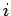flowing through the inductor from zero to some final value. As the current through the inductor is ramped up, an emf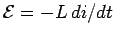is generated, which acts to oppose the increase in the current. Clearly, work must be done against this emf by the voltage source in order to establish the current in the inductor. The work done by the voltage source during a time interval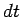is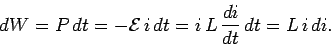(247)

Here,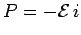is the instantaneous rate at which the voltage source performs work. To find the total work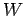done in establishing the final currentin the inductor, we must integrate the above expression. Thus,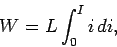(248)

giving(249)

This energy is actually stored in the magnetic field generated by the current flowing through the inductor. In a pure inductor, the energy is stored without loss, and is returned to the rest of the circuit when the current through the inductor is ramped down, and its associated magnetic field collapses.

Consider a simple solenoid. Equations  (244), (246), and (249) can be combined to give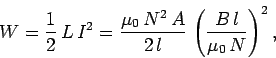(250)

which reduces to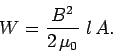(251)

This represents the energy stored in the magnetic field of the solenoid. However, the volume of the field-filled core of the solenoid is, so the magnetic energy density (i.e., the energy per unit volume) inside the solenoid is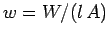, or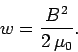(252)

It turns out that this result is quite general. Thus, we can calculate the energy content of any magnetic field by dividing space into little cubes (in each of which the magnetic field is approximately uniform), applying the above formula to find the energy content of each cube, and summing the energies thus obtained to find the total energy.

When electric and magnetic fields exist together in space, Eqs. (122) and (252) can be combined to give an expression for the total energy stored in the combined fields per unit volume: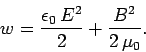(253)Next: The Circuit Up: Inductance Previous: Self Inductance
Richard Fitzpatrick 2007-07-14